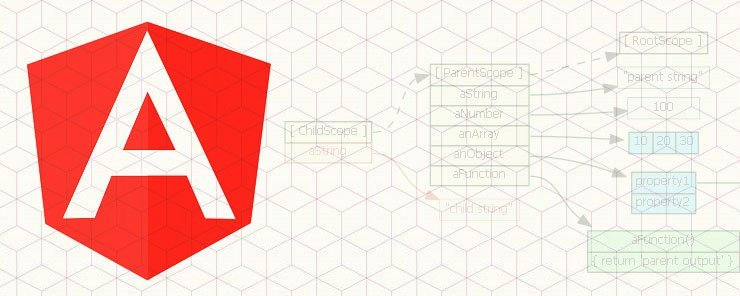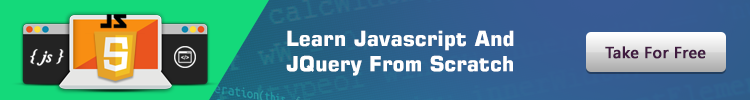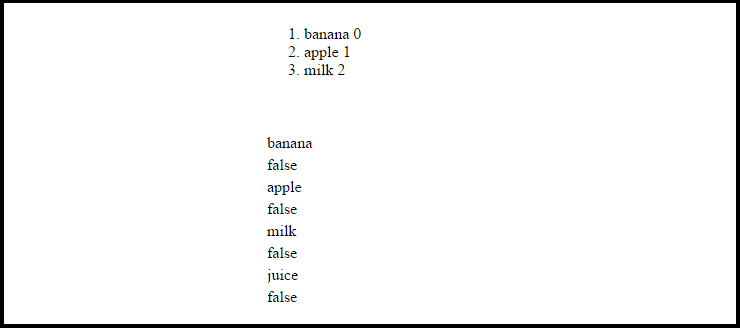# Learn about ng-repeat directive in AngularJS

0
5062In the previous tutorials, we’ve learned about the scope element as well as controllers. In this lesson we’ll go over the ng-repeat directive. ng-repeat recreates the same HTML element once for each item in an array. The best way to learn how it work is by using an example. We’ll start by creating an Angular app and set up the controller in the script:

```<script>
var app1 = angular.module('app1', []);
app1.controller('Grocery', function(\$scope) {
\$scope.lst = [
{item:'banana', needed:false},
{item: 'apple', needed:false},
{item:'milk', needed:false},
{item:'juice', needed:false}
]
});

</script>
```

We have created a grocery list array. It’s an array of objects, each containing a key-value pair(1 string, 1 boolean). Let‘s move on to the HTML and display our grocery list:

```<body ng-app='app1'>
<div ng-controller = 'Grocery'>
<ol>
<li ng-repeat='x in lst'>
{{x.item}} {{\$index}}
</li>
</ol>
</div>
</body>
```

Explanation: We have printed out each and every one of the grocery items and its array index using expressions. ‘X in 1st’ means we’re iterating over each of the 1st array items, like in a loop. We could put ‘Y’ or ‘grocery’ instead of ‘x’, it doesn’t matter what we call it. Since it’s an array of objects, we specify the key to get its value, while treating ‘x’ as if it were the object’s name (or the name of each object in the array).

One of the few things we can add with ng-repeat are an option to display only items of a certain index. For example, if we want to display all array items except the last one, we can use ng-if with \$index, like so:

```<div ng-controller = 'Grocery'>
<ol>
<li ng-repeat='x in 1st' ng-if='\$index < 3'>
{{x.item}} {{\$index}}
</li>
</ol>
</div>
```

As we’re cycling through the array items, we can use \$first which would return true if the current item is the first item in the array. We can use \$last which would return true if the current item is the last item. We can use \$middle which returns true if the current item is neither the first nor the last.We can also use \$even which returns true if the current item has an even index and \$odd which returns true if the current item has an odd index.

We can also use ng-repeat-start and ng-repeat-end to use ng-repeat on two elements rather than one. Let’s see an example of that:

```<table>
<tr ng-repeat-start='x in 1st'>
<td>
{{x.item}}
</td>
<tr ng-repeat-end>
<td>
{{x.needed}}
</td>
</tr>
</table>
```

Let’s run the full code and see the outcome:

```<body ng-app='app1'>
<div ng-controller = 'Grocery'>
<table>
<tr ng-repeat-start='x in 1st'>
<td>
{{x.item}}
</td>
<tr ng-repeat-end>
<td>
{{x.needed}}
</td>
</tr>
</table>
</div>
<script>
var app1 = angular.module('app1', []);
app1.controller('Grocery', function(\$scope) {
\$scope.lst = [
{item:'banana', needed:false},
{item: 'apple', needed:false},
{item:'milk', needed:false},
{item:'juice', needed:false}
]
});
</script>
</body>
```In this AngularJS tutorial, we’ve gotten acquainted with the ng-repeat directive. The ng-repeat can iterate over array elements in a loop-like manner. We use ‘x in ArrayName’ to refer to each item in the array/collection. With ng-repeat, we can also use a group of boolean variables and various conditional statements to display or select/exclude only items with a certain kind of index.